Trial ends in

Chapter 2

## Thermodynamics and Chemical Kinetics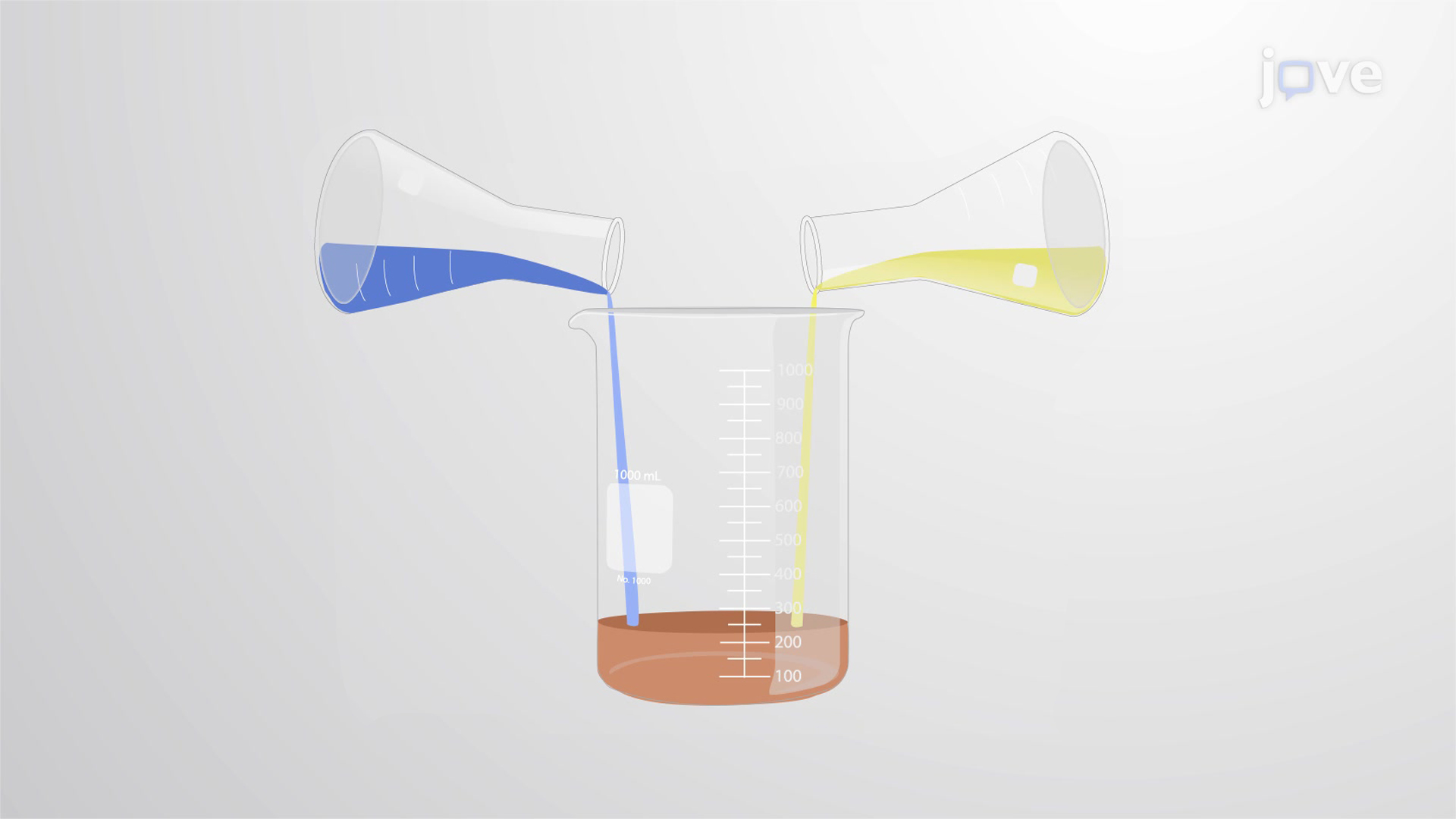A balanced chemical equation provides the information of chemical formulas of the reactants and products involved in the chemical change. A…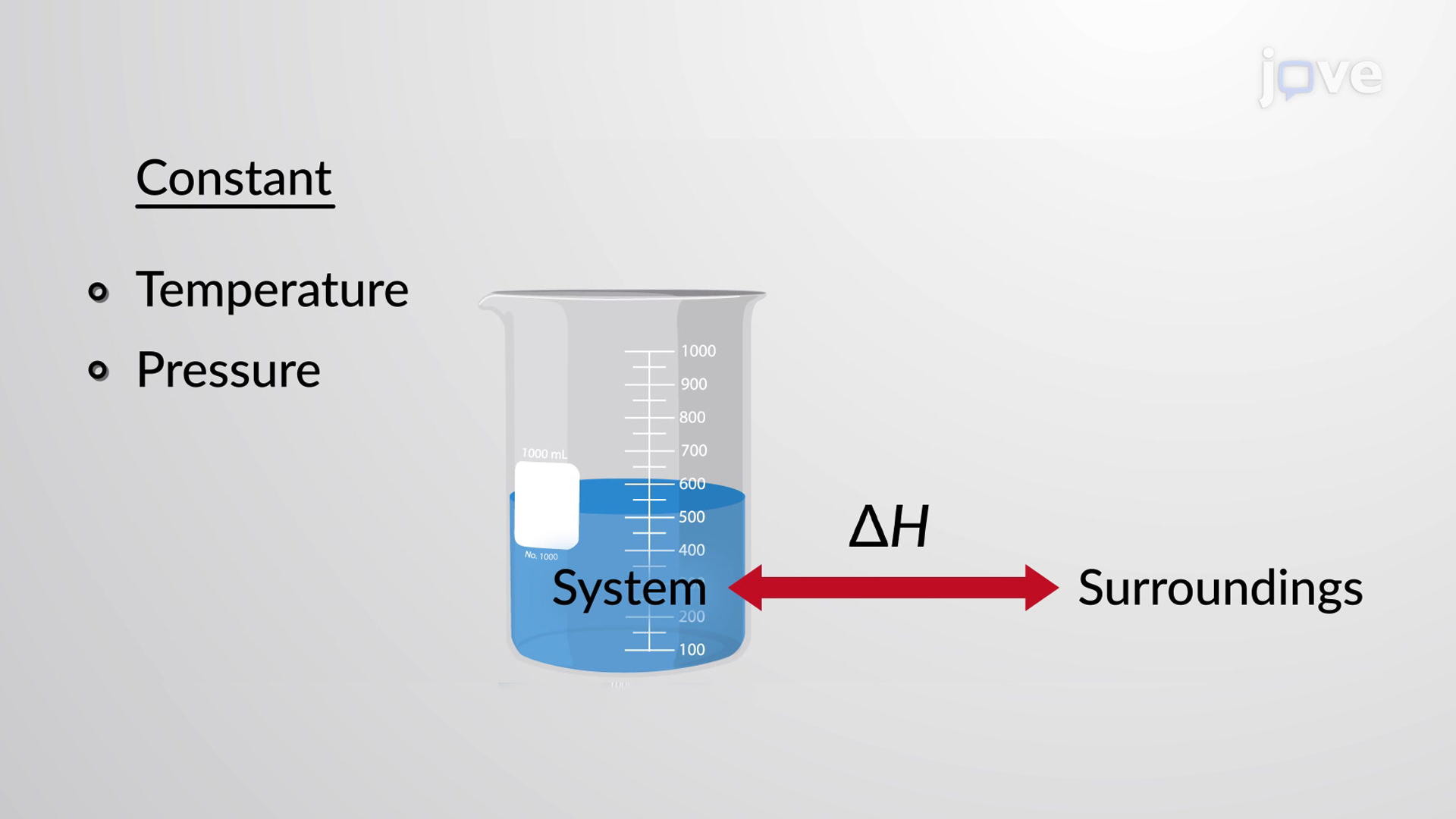Combustion, commonly known as burning, is a reaction in which a substance reacts with an oxidizing agent, which in most cases is molecular oxygen, to…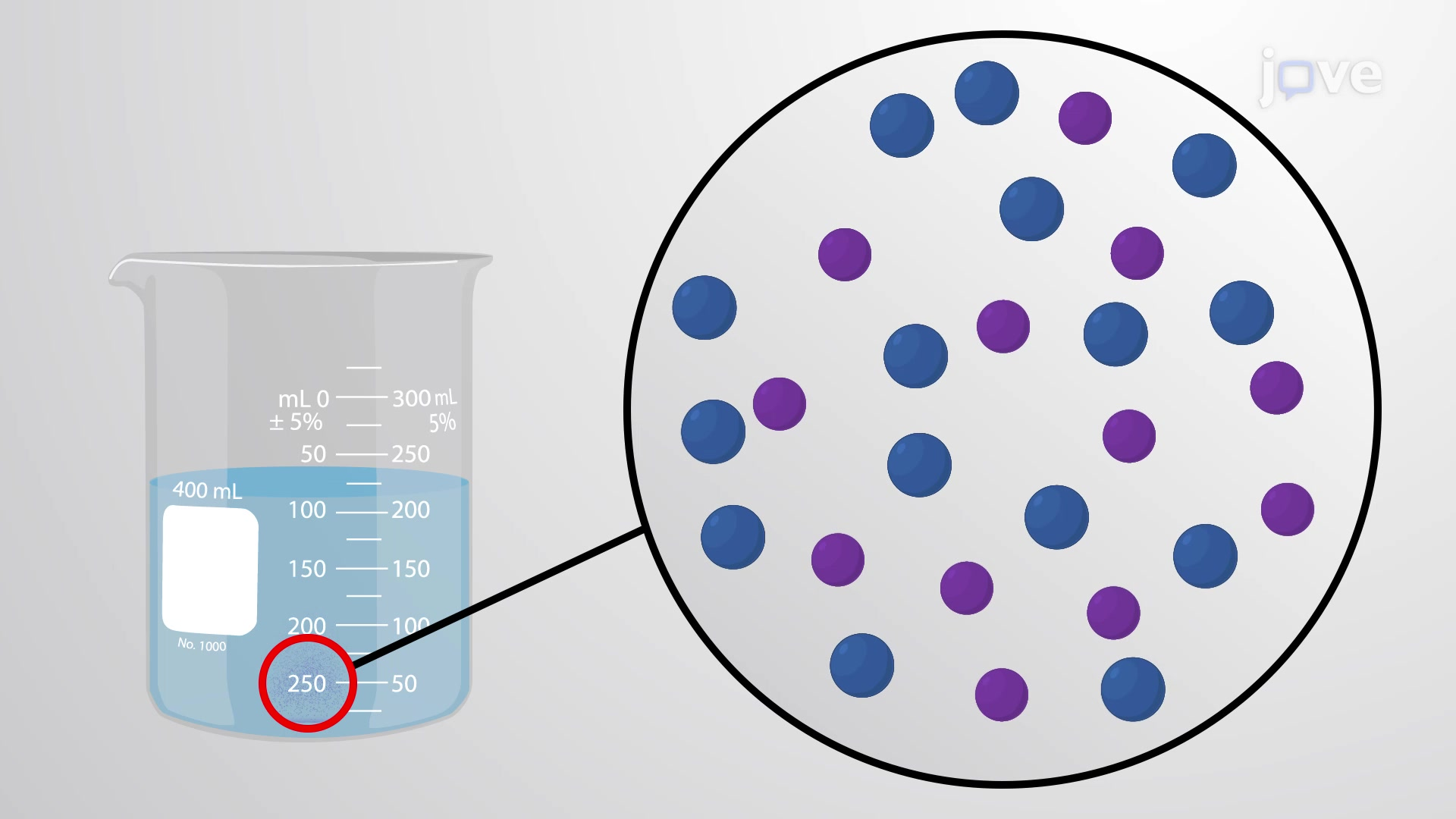The formation of a solution is an example of a spontaneous process, which is a process that occurs under specified conditions without energy from…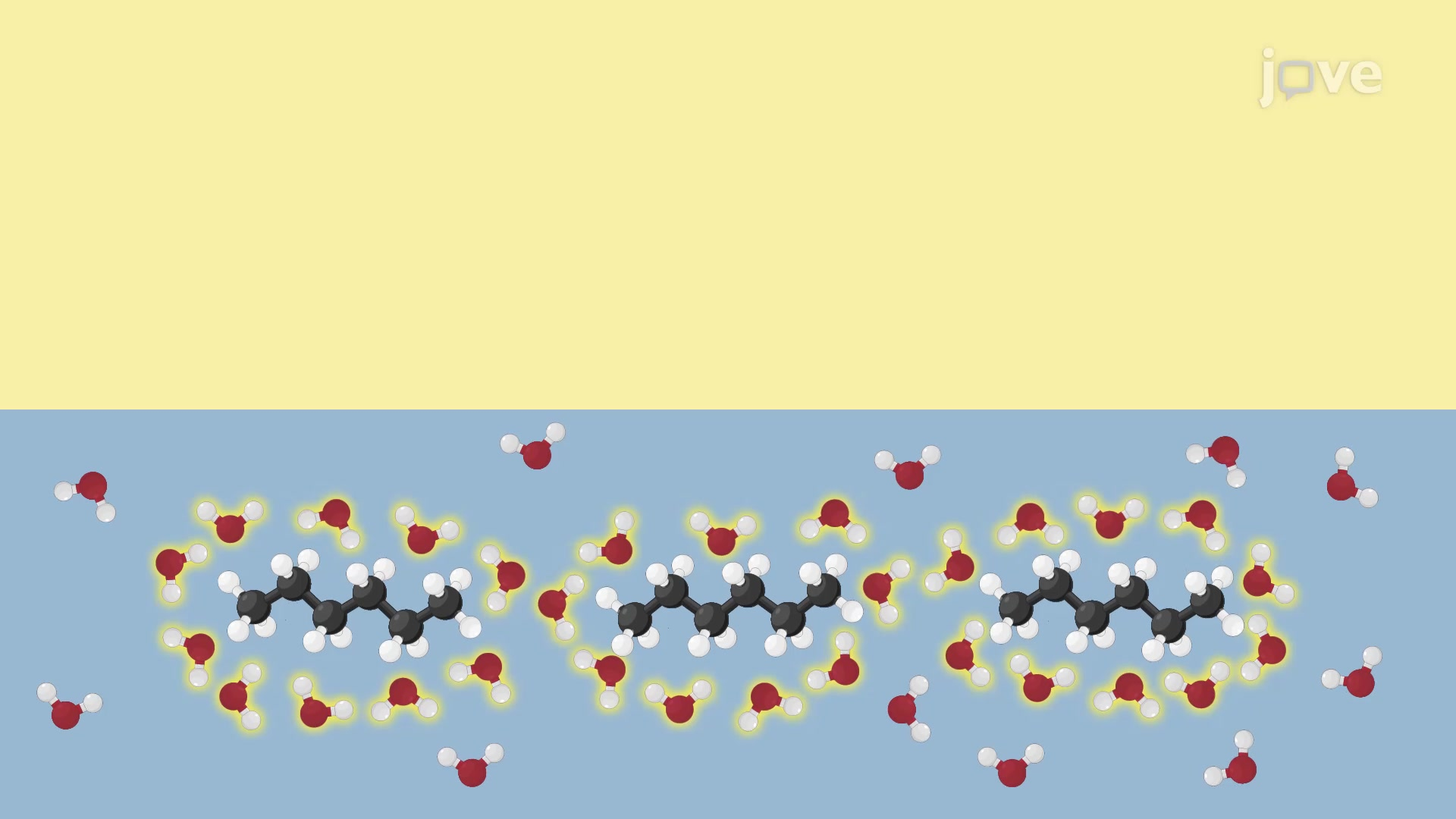The process of surrounding a solute with solvent is called solvation. It involves evenly distributing the solute within the solvent. The rule of…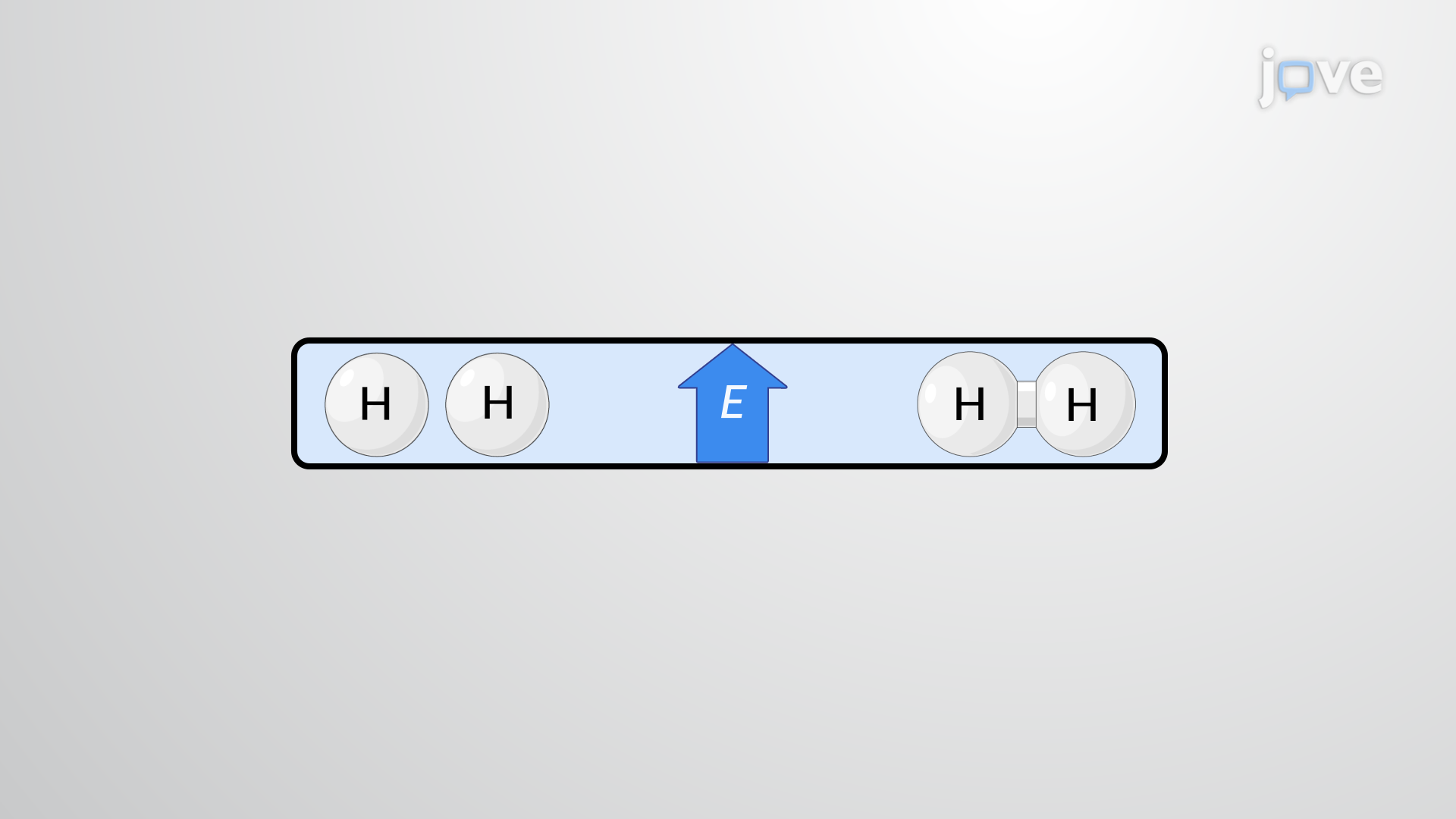The spontaneity of a process depends upon the temperature of the system. Phase transitions, for example, will proceed spontaneously in one direction…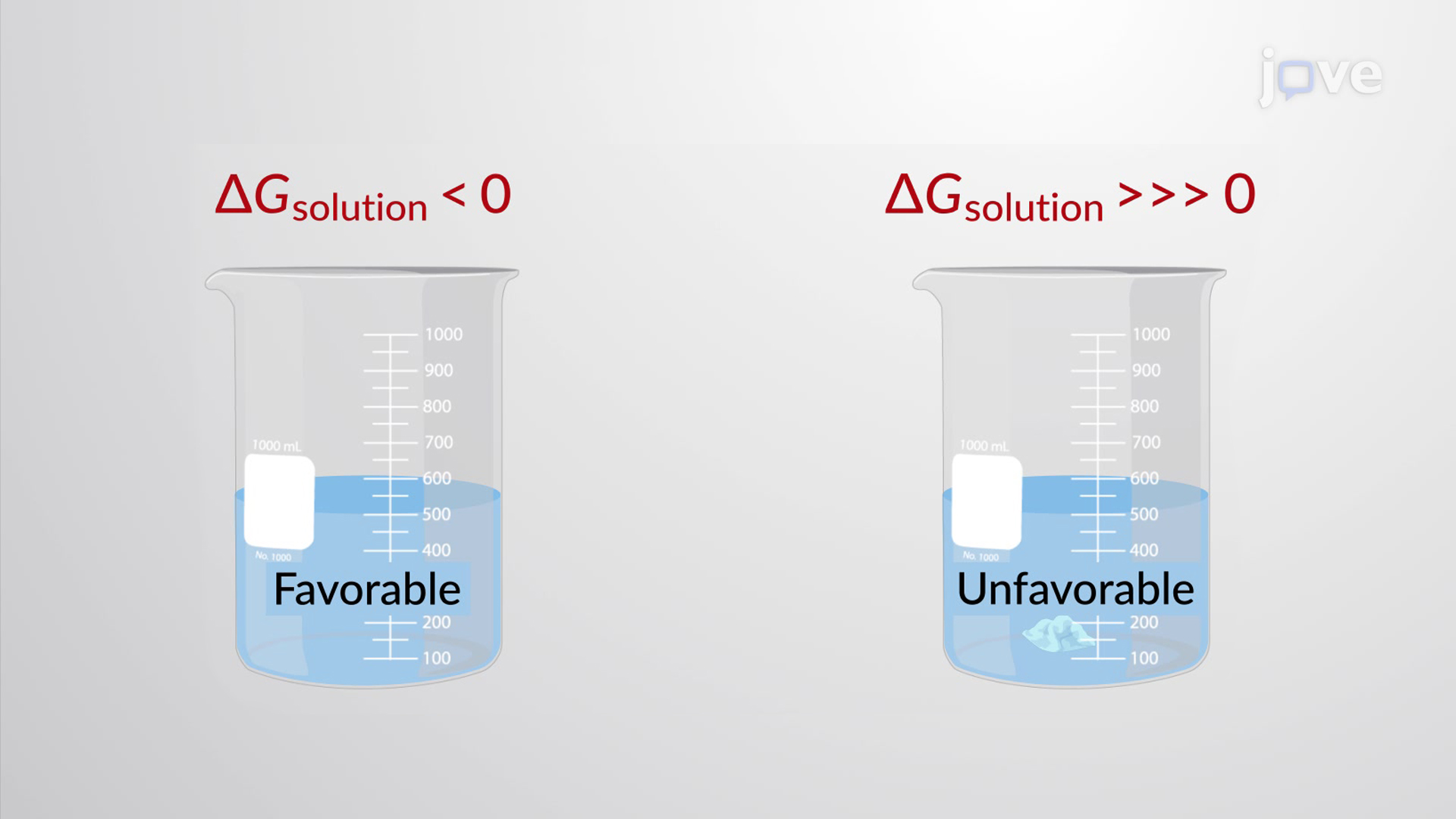The free energy change associated with dissolving a solute in a liter of solvent is called the free energy of a solution, ΔGsolution. The…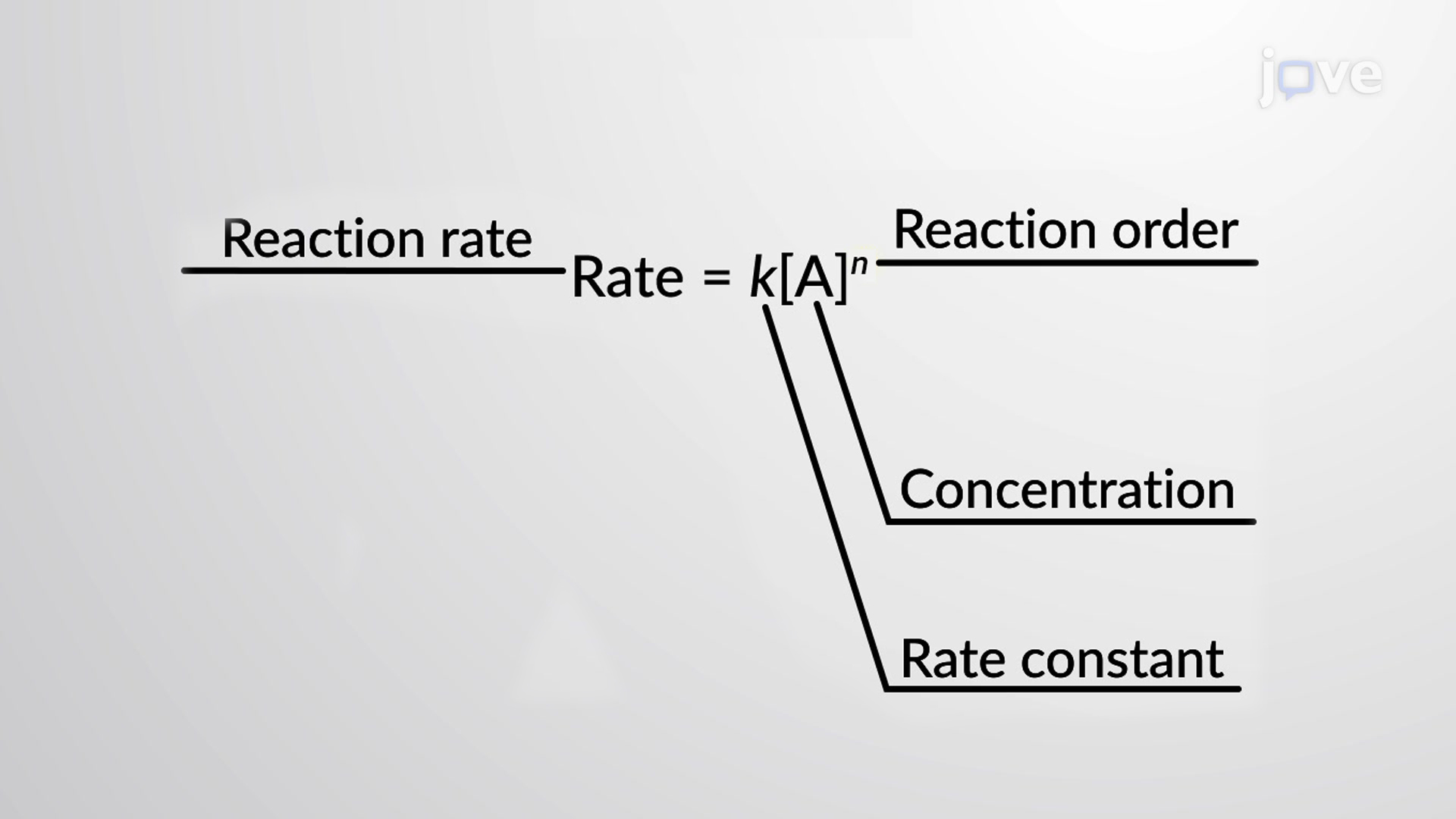The rate of a reaction is affected by the concentrations of reactants. Rate laws (differential rate laws) or rate equations are mathematical…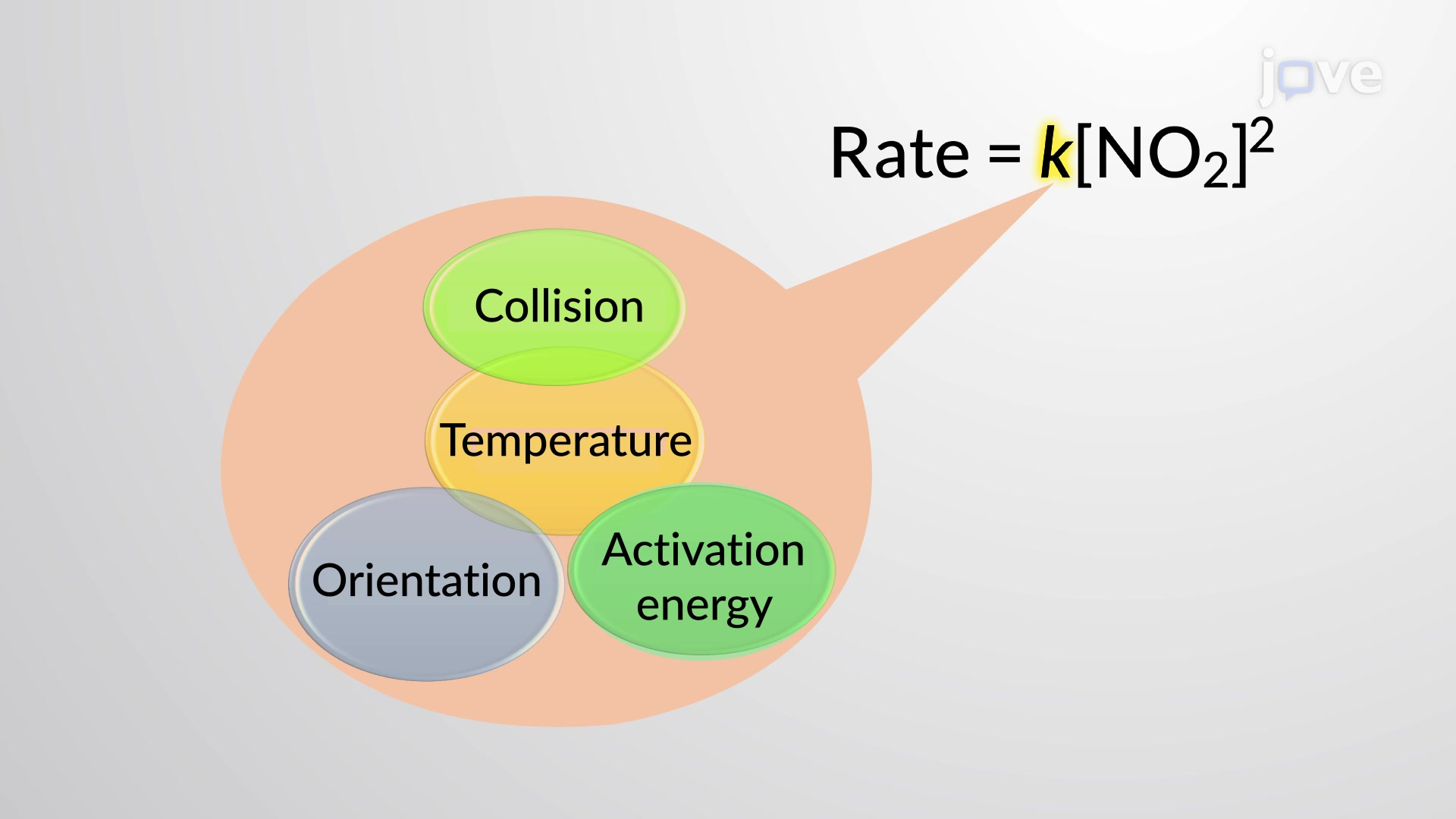The Arrhenius equation, relates the activation energy and the rate constant, k, for many chemical reactions. In this equation, R is the ideal gas…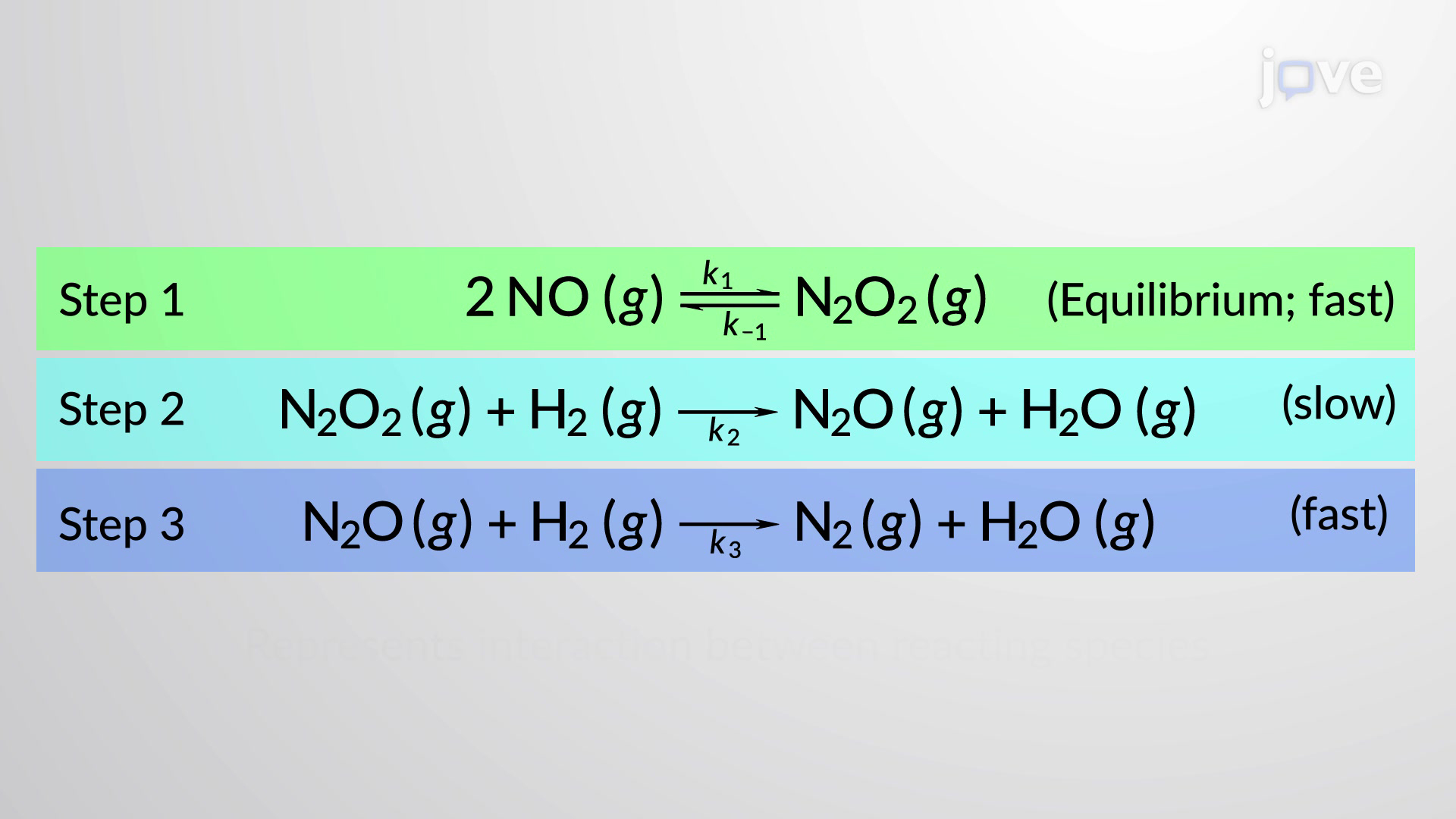Chemical reactions often occur in a stepwise fashion involving two or more distinct reactions taking place in a sequence. A balanced equation…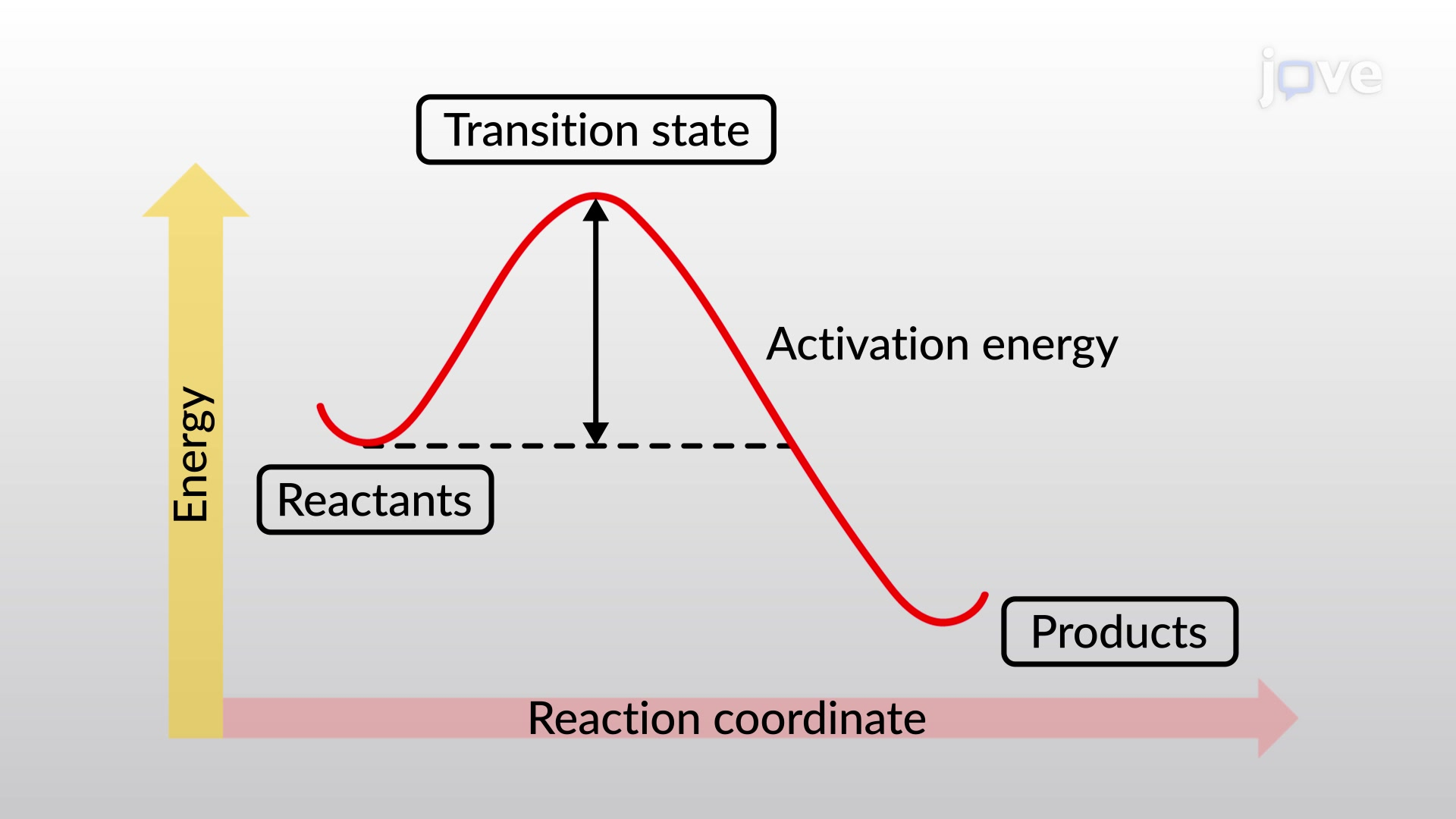Bond energy is the energy required to break a bond homolytically. These values are usually expressed in units of kcal/mol or kJ/mol and are referred…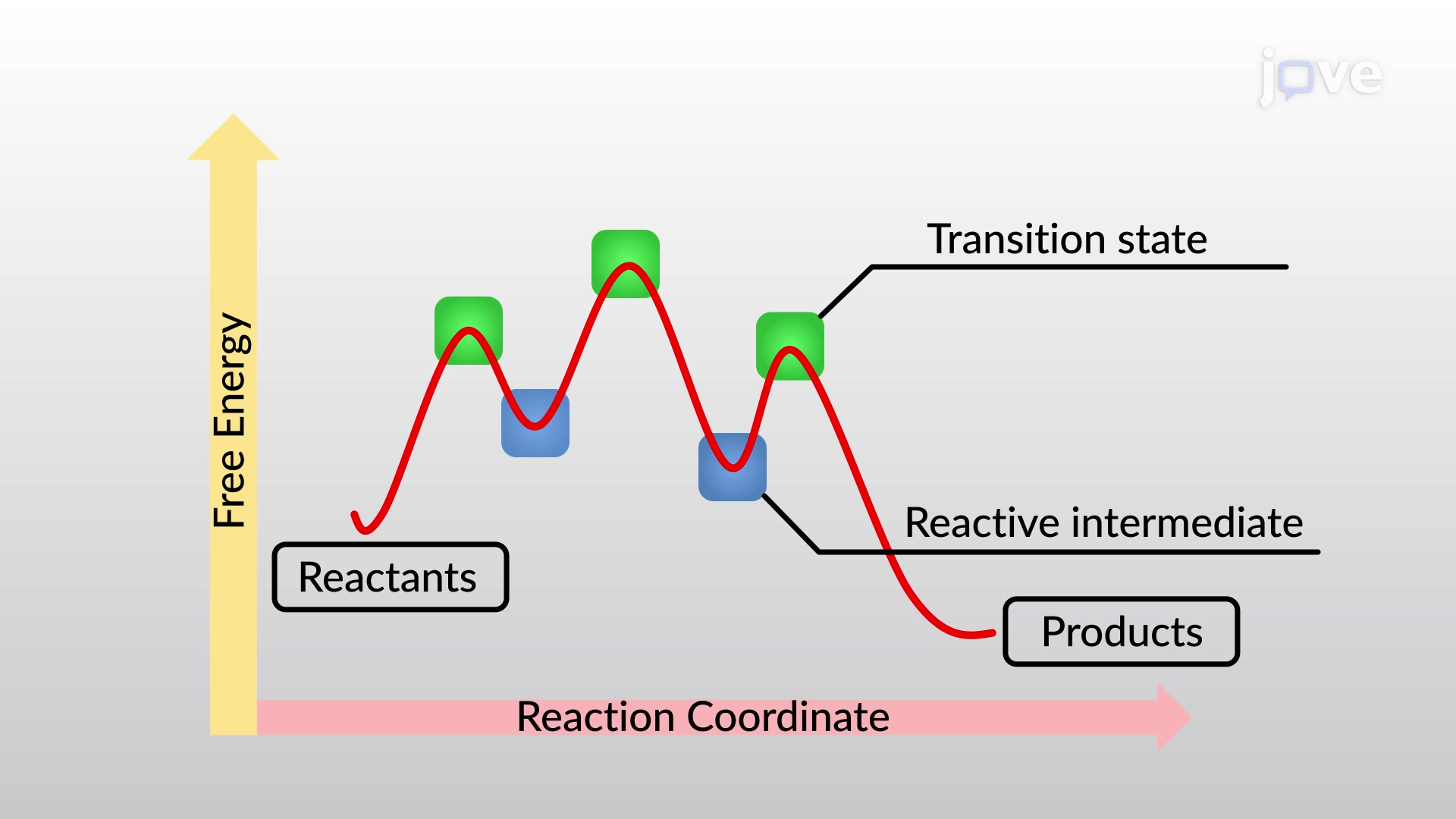Free-energy diagrams, or reaction coordinate diagrams, are graphs showing the energy changes that occur during a chemical reaction. The reaction…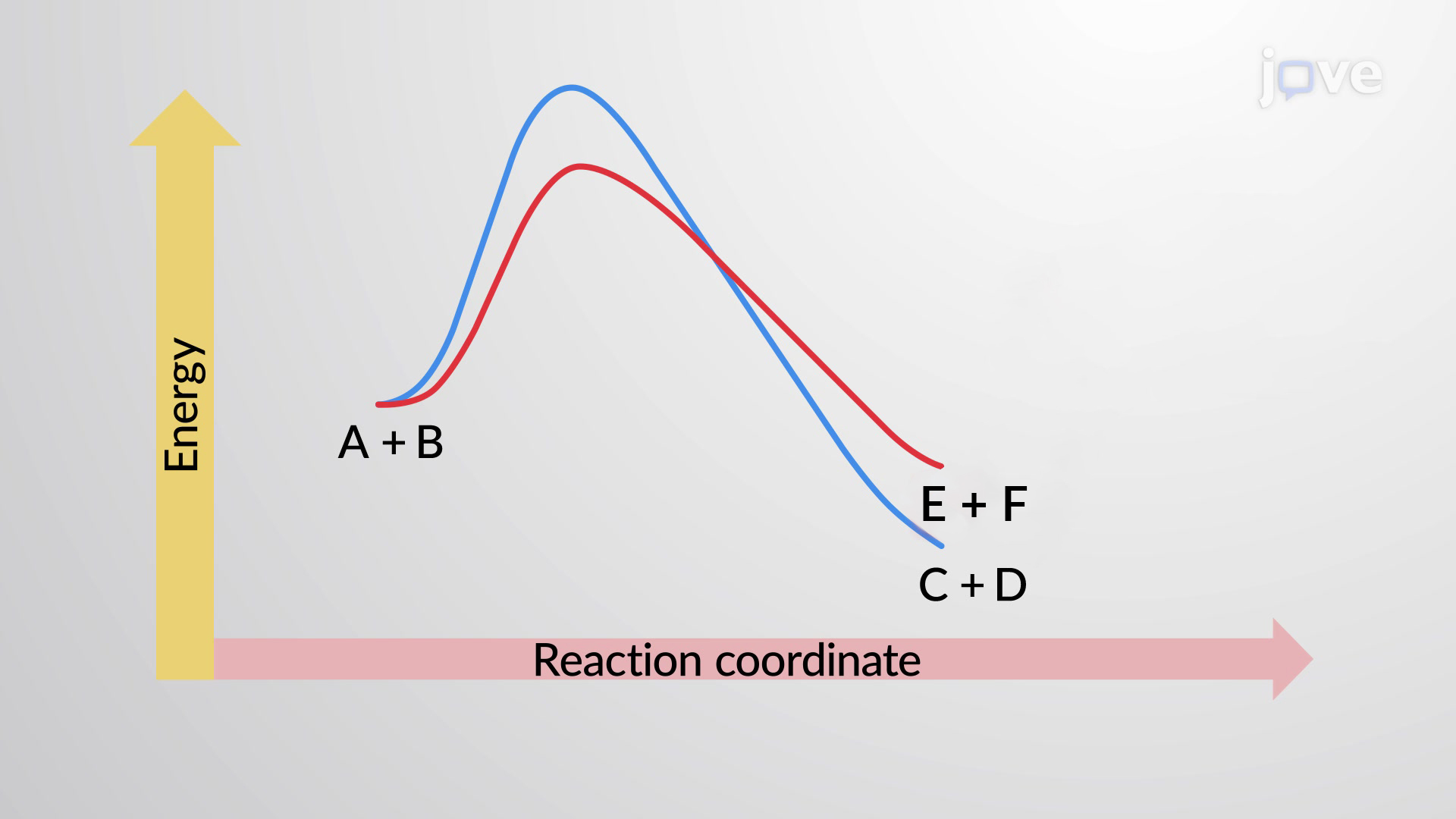Kinetics describes the rate and path by which a reaction occurs. In contrast, thermodynamics deals with state functions and describes the properties,…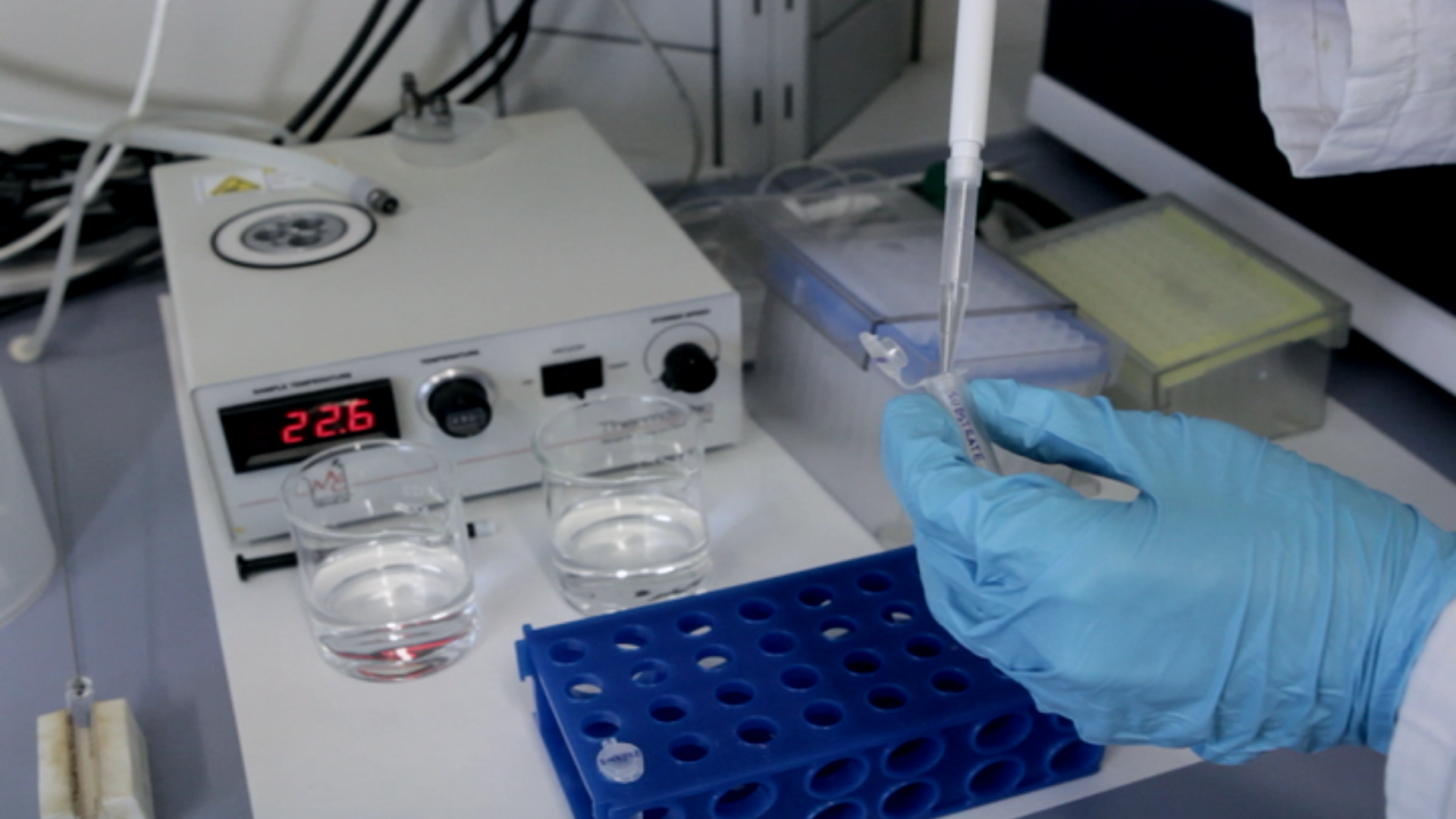Isothermal titration calorimetry (ITC) is a well-described technique that measures the heat released or absorbed during a chemical reaction, using it…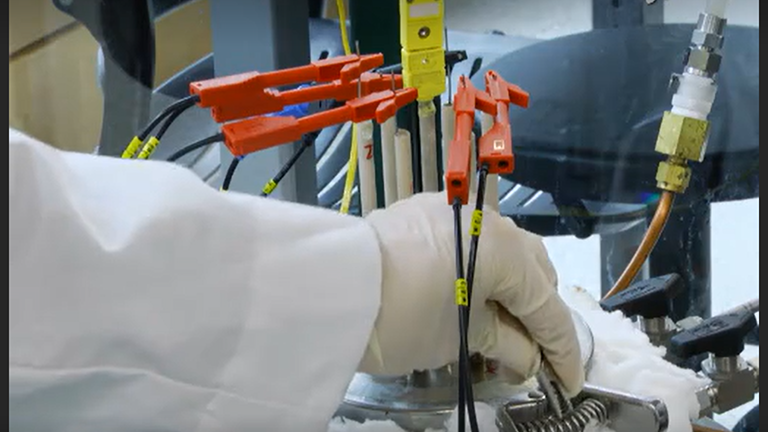A novel electrochemical cell based on a CaF2 solid-state electrolyte has been developed to measure the electromotive force (emf) of binary alkaline…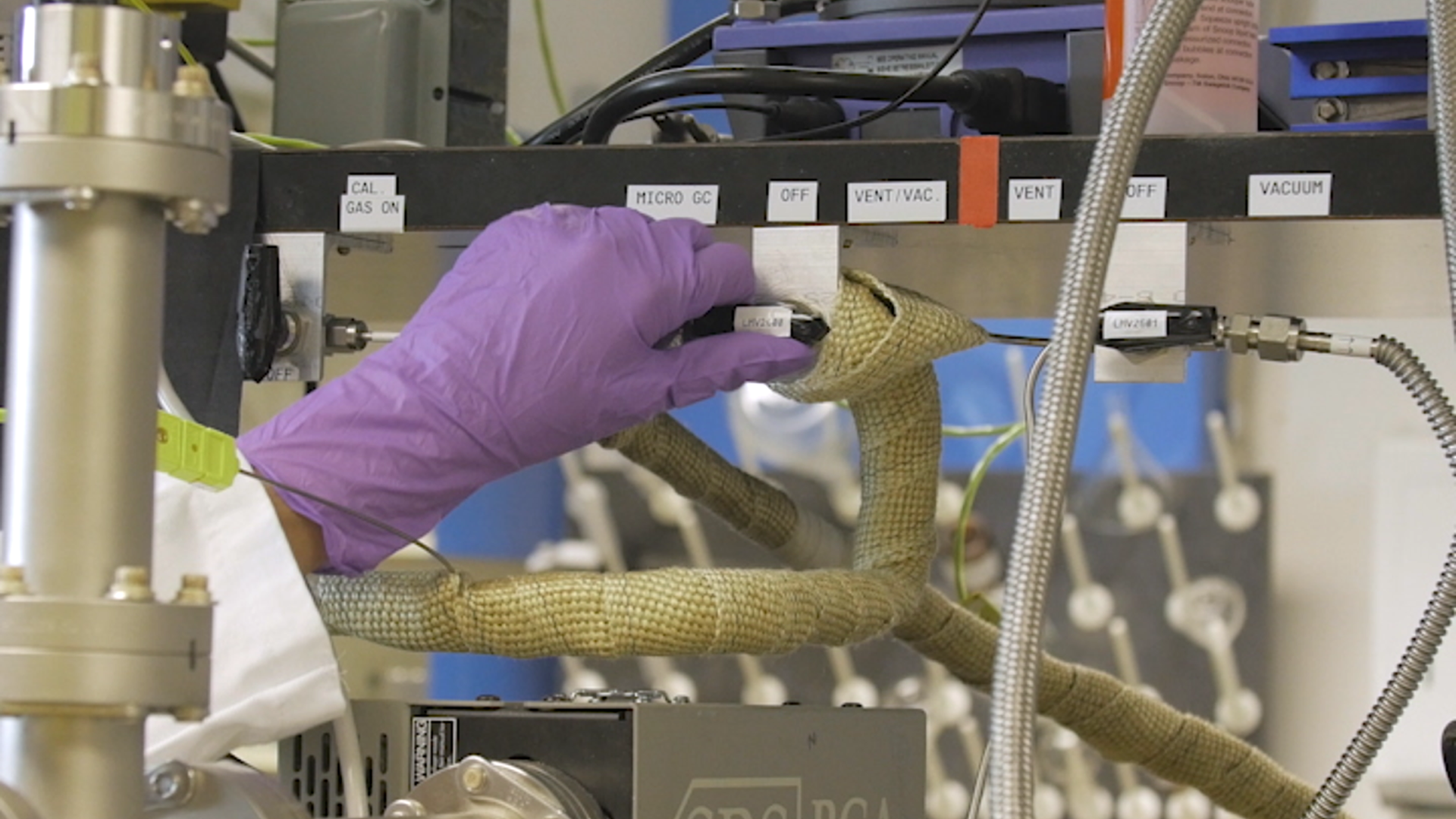Temperature programmed reaction (TPRxn) is a simple yet powerful tool for screening solid catalyst performance at a variety of conditions. A TPRxn…

### Get cutting-edge science videos from JoVE sent straight to your inbox every month.X